Saturday, June 12, 2021
Home > download jstse papers > JSTSE PREVIOUS YEAR QUESTION PAPER 2008-09

# JSTSE PREVIOUS YEAR QUESTION PAPER 2008-09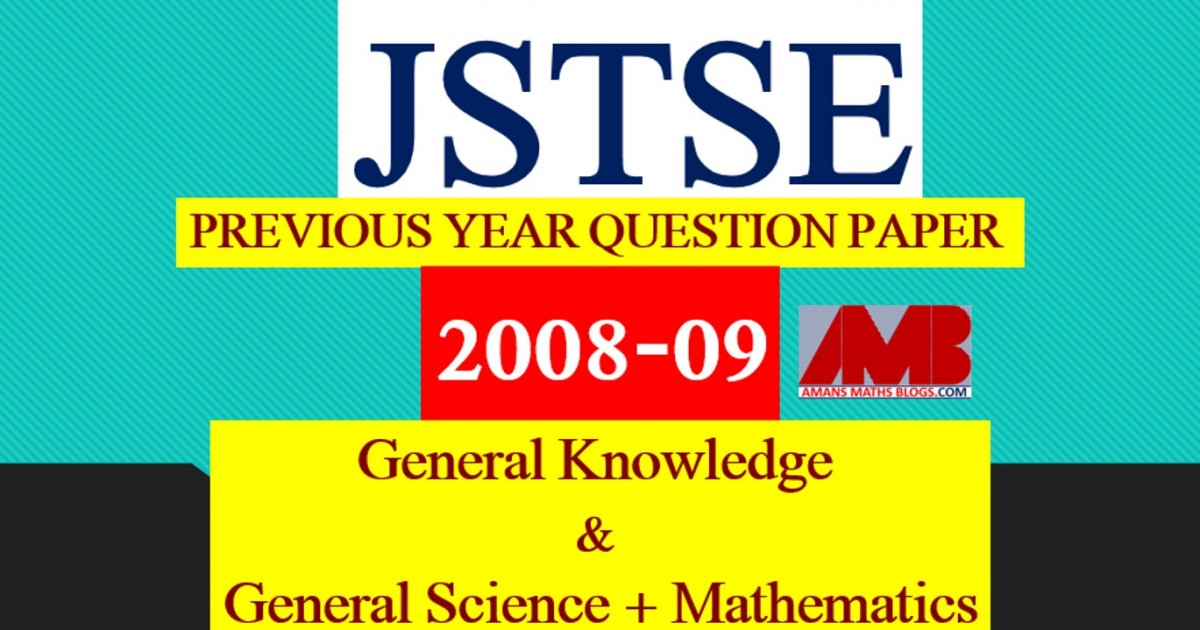# JSTSE PREVIOUS YEAR QUESTION PAPER 2008-09

In this post, you will get JSTSE Previous Year Question Paper 2008-09.

Junior Science Talent Search Examination (JSTSE)
Junior Science Talent Search Examination (JSTSE) is conducted by Science Branch of Dte. Of Education, Delhi every year usually in the month of January for the students of recognised schools of Delhi.
Eligibility: – Any student of recognised school studying in Class IX in Govt./Govt. Aided/Public/KV/Navodaya/NDMC and must have secured 65% marks in class VIII.

Examination fee: – No examination fee is charged from students for appearing in this exam.
Type of Examination: – The examination consists of two papers.
I. General Knowledge
No. of Questions: 50       Marks : 50     Time Alloted: 50 min
50 50 50 min.
II . General Science and Mathematics
No. of Questions : 150      Marks : 150     Time Alloted: 150 min

No. of Scholarships:- There are 150 scholarships for JSTSE per year.

JSTSE / MVPP Previous Year Questions Papers with Solutions
JSTSE 2020-21 Question Papers with Answer Keys & Solutions
JSTSE 2019-20 Question Papers with Answer Keys & Solutions
JSTSE 2018-19 Question Papers with Answer Keys & Solutions
JSTSE 2017-18 Question Paper Not Available
JSTSE 2016-17 Question Papers with Answer Keys & Solutions
JSTSE 2015-16 Question Papers with Answer Keys & Solutions
JSTSE 2014-15 Question Papers with Answer Keys & Solutions
JSTSE 2013-14 Question Papers with Answer Keys & Solutions
JSTSE 2012-13 Question Papers with Answer Keys & Solutions
JSTSE 2011-12 Question Papers with Answer Keys & Solutions
JSTSE 2010-11 Question Papers with Answer Keys & Solutions
JSTSE 2009-10 Question Papers with Answer Keys & Solutions
JSTSE 2008-09 Question Papers with Answer Keys & Solutions
JSTSE 2007-08 Question Papers with Answer Keys & Solutions
JSTSE 2006-07 Question Papers with Answer Keys & Solutions
JSTSE Physics Questions with Answer Keys & Solutions
JSTSE Chemistry Question with Answer Keys & Solutions
JSTSE Biology Question with Answer Keys & Solutions
JSTSE Maths Question with Answer Keys & Solutions

## General Science + Mathematics

Click Here To Download This File

JSTSE Previous Year Question Papers 2008-09 Ques No 1:

If x = 21/3 + 22/3, then the value of x3 will be

Options:

A. 6 + 6x

B. 8

C. 6

D. 6x

JSTSE Previous Year Question Papers 2008-09 Ques No 2:

If x + 1/x = 4, then the value of x3 – x2 – 1/x3 – 1/x2 will be

Options:

A. 64

B. 48

C. 38

D. 0

JSTSE Previous Year Question Papers 2008-09 Ques No 3:

On evaluating (1.1)3 – (0.7)3 – (0.4)3, we get

Options:

A. 0

B. 0.924

C. 1.174

D. 8.8

JSTSE Previous Year Question Papers 2008-09 Ques No 4:

On simplifying [(a2 – b2)3(b2 – c2)3 + (c2 – a2)3] / [(a – b)3 + (b – c)3 + (c – a)3]

Options:

A. 0

B. 3(a + b)(b + c)(c + a)

C. 3(a – b)(b – c)(c – a)

D. (a + b)(b + c)(c + a)

JSTSE Previous Year Question Papers 2008-09 Ques No 5:

(0.83 x 0.83 x 0.83 + 0.17 x 0.17 x 0.17) / (0.83 x 0.83 – 0.83 x 0.17 + 0.17 x 0.17) =

Options:

A. 0

B. 1

C. (0.83 – 0.17)3

D. 0.83 + 0.83 x 0.17

JSTSE Previous Year Question Papers 2008-09 Ques No 6:

If R1 and R2 are the remainders when polynomials x3 + 2x2 – 5ax – 7 and x3 + ax2 – 12x + 6 are divided by x + 1 and x – 2 respectively, If R1 – R2 = 0, then the value of a is

Options:

A. -4

B. -1

C. 13

D. -13

JSTSE Previous Year Question Papers 2008-09 Ques No 7:

If x, y, z, are positive real numbers, then the value of √(x-1y) √(y-1z) √(z-1x) will be

Options:

A. xyz

B. x-1y-1z-1

C. 1

D. 0

JSTSE Previous Year Question Papers 2008-09 Ques No 8:

If 1/x = 2, 1/y = 3, 1/z = 4, then the value of (x-2 + y-2 + z-2)-1 will be

Options:

A. (1/9)-1

B. (1/19)-1

C. (1/29)-1

D. 1/29

JSTSE Previous Year Question Papers 2008-09 Ques No 9:

In the given figure, AB || CD, ∠EAB = 120 degree and ∠ECB = 100 degree. Then the value of ∠AEC isOptions:

A. 140 degree

B. 120 degree

C. 100 degree

D. 20 degree

JSTSE Previous Year Question Papers 2008-09 Ques No 10:

The bisector of base angles ∠B and ∠C of a ∆ABC encloses an angle of 135 degree, then angle A will be

Options:

A. 45 degrees

B. 62.5 degrees

C. 90 degrees

D. None of these

JSTSE Previous Year Question Papers 2008-09 Ques No 11:

AD is the median of ∆ABC and DE is median of ∆ABD. If ar(∆ABC) = 40 cm2, then the value of ar(∆BDE) is

Options:

A. 13.33 cm2

B. 20 cm2

C. 10 cm2

D. None of these

JSTSE Previous Year Question Papers 2008-09 Ques No 12:

ABCD is a parallelogram X and Y are mid points of BC and CD respectively, then the ar(CYX) will be

Options:

A. (1/4) ar(ABCD)

B. (3/4) ar(ABCD)

C. (1/8) ar(ABCD)

D. (3/8) ar(ABCD)

JSTSE Previous Year Question Papers 2008-09 Ques No 13:

In a parallelogram ABCD, AE ⊥ DC and CF ⊥ AD. If AB = 16 cm, AE = 8 cm, and CF = 10 cm, then AD will be

Options:

A. 12.8 cm

B. 20 cm

C. 18 cm

D. 5 cm

JSTSE Previous Year Question Papers 2008-09 Ques No 14:

In the given figure, OCDE is a rectangle inscribed in a quadrant of a circle of radius 10 cm. If OE = 2√5, then the area of rectangle isOptions:

A. 20√5 cm2

B. 20 cm2

C. 40 cm2

D. 40√5 cm2

JSTSE Previous Year Question Papers 2008-09 Ques No 15:

If two concentric circle with centre O have A, B, C, D as points of intersections with L as shown in figure, If AD = 14 cm, BC = 10 cm, then the value of AB will beOptions:

A. 2 cm

B. 4 cm

C. 5 cm

D. 7 cm

JSTSE Previous Year Question Papers 2008-09 Ques No 16:

An equilateral triangle of side 9 cm is inscribed in a circle. The inradius of the circle is

Options:

A. 9 cm

B. 4.5 cm

C. 3√3 cm

D. 3 cm

JSTSE Previous Year Question Papers 2008-09 Ques No 17:

In the figure, O is the centre of the circle ∠OAB = 20 degree and ∠OCB = 55 degree, then ∠BOC will be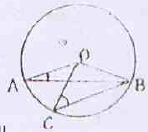Options:

A. 55 degree

B. 70 degree

C. 75 degree

D. 95 degree

JSTSE Previous Year Question Papers 2008-09 Ques No 18:

In the figure, O is the centre of the circle. The value of ∠CBD is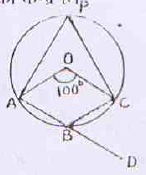Options:

A. 100 degree

B. 75 degree

C. 50 degree

D. 80 degree

JSTSE Previous Year Question Papers 2008-09 Ques No 19:

In the figure, O is the centre of the circle. ACBO is a parallelogram, then the value of x is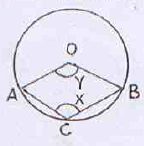Options:

A. 90 degree

B. 2y

C. (180 – y) degree

D. 120 degree

JSTSE Previous Year Question Papers 2008-09 Ques No 20:

A metallic sheet of the rectangular shape with dimension 35 cm x 20 cm. From each one of its corner, a square of 5 cm is cut off and open box is made of remaining sheet. The volume of the box is

Options:

A. 1250 cm3

B. 2250 cm3

C. 3000 cm3

D. None of these

JSTSE Previous Year Question Papers 2008-09 Ques No 21:

A semi-circular sheet of metal of diameter 28 cm is bent into on open conical cup. The depth of the cup is

Options:

A. 14 cm

B. 14√3 cm

C. 7 cm

D. 7√3 cm

JSTSE Previous Year Question Papers 2008-09 Ques No 22:

The following observation have been arranged in ascending order 29, 32, 48, 50, x, x+2, 72, 78, 84, 95. If median of the data is 63. Then the value of x is

Options:

A. 61

B. 62

C. 63

D. 64

JSTSE Previous Year Question Papers 2008-09 Ques No 23:

If M is the mean of n observations x1, x2, x3, …, xn, then the value of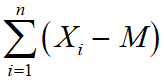Options:

A. 0

B. 1

C. M

D. 2M

JSTSE Previous Year Question Papers 2008-09 Ques No 24:

If the mean of 10 observations is 61.4 and median is 63, then the mode will be

Options:

A. 63

B. 64.6

C. 66.2

D. 62.2

JSTSE Previous Year Question Papers 2008-09 Ques No 25:

The percentage of marks obtained by a students in the monthly unit test are given below. The probability that the students gets at least 60% marks is

Options:

A. 0

B. 2/3

C. 3/5

D. 4/5

error: Content is protected !!